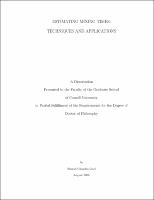## Estimating Mixing Times: Techniques and Applications##### Abstract
How many times do you have to shuffle a deck of n cards before it is close to random? log n? n? n^3? Similar convergence rate questions for finite Markov chains are central to solving applied problems in diverse fields including physics, computer science and biology. This thesis investigates two general techniques for estimating mixing times for finite Markov chains: modified logarithmic Sobolev inequalities and Faber-Krahn inequalities; and analyzes the convergence behavior of a specific family of random walks: the top to bottom shuffles. Logarithmic Sobolev inequalities are a well-studied technique for estimating convergence rates for Markov chains. In contrast to continuous state spaces, there are several distinct modified log Sobolev inequalities in the discrete setting. Here we derive modified log Sobolev inequalities for several models of random walk, including the random transposition shuffle. These results lead to tight mixing time estimates, and additionally, yield concentration inequalities. Faber-Krahn inequalities have been used to estimate the rate of decay of the heat kernel on complete, non-compact manifolds and infinite graphs. We develop this technique in the setting of finite Markov chains, proving upper and lower mixing time bounds via the spectral profile. This approach lets us recover previous conductance-based bounds of mixing time, and in general leads to sharper estimates of convergence rates. We apply this method to several models, including groups with moderate growth, the fractal-like Viscek graphs, and the torus, and obtain tight bounds on the corresponding mixing times. A deck of n cards is shuffled by repeatedly moving the top card to one of the bottom k positions of the deck uniformly at random. We give upper and lower bounds on the total variation mixing time for this shuffle as k ranges from a constant to n. We also consider a symmetric variant of this walk which at each step either inserts the top card randomly into the bottom k positions or moves a random card from the bottom k positions to the top. For this reversible shuffle we derive L^2 mixing time bounds.